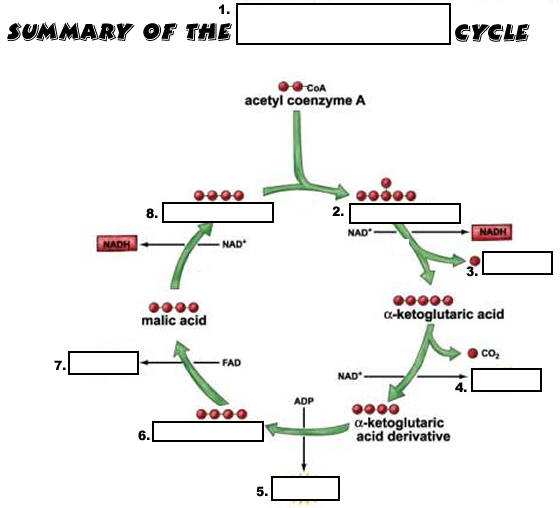Cell Respiration PPT Questions – B1

PowerPoint Question Guide

1. _______ is the energy used by all cells.

2. ATP stands for ________________ ______________.

3. ATP is an ___________ molecule containing high-energy ____________ bonds.

4. The sugar in ATP is ____________, while the nitrogen base is ___________.

5. How many phosphate groups does ATP contain?

6. How do we get energy from ATP?

7. Make a simple sketch of ATP and show the high-energy bond that is broken.

8. To break the last phosphate bond in ATP, _________ must be added.

9. The process is called ___________.

10. What enzyme is used to help weaken & break the last phosphate bond in ATP?

12. When the last phosphate bond of ATP is broken, __________ and a free __________ form.

13. What enzyme can be used to rejoin ADP and a free phosphate to make more ATP?

14. Using ATP’s energy and then remaking it is called the ________________ cycle.

15. In the body, ATP is made during the process of _____________ ________________.

16. Cellular respiration takes place in both ____________ and ____________.

17. Cellular respiration requires the gas ____________.

18. In cellular respiration, _____________ is oxidized (loses electrons) and ___________ is reduced (gains electrons).

19. The breakdown of one glucose molecule results in ________ to _______ ATP molecules of energy.

20. Write the overall equation for cellular respiration.

21. Cellular respiration is an example of a ________ reaction.

22. REDOX stands for _____________-____________ reactions.

23. What are the products of cellular respiration?

24. What carries the energized electrons from glucose in cellular respiration?

25. NAD+_ is a _____________ that forms ____________ when it is reduced (picks up electrons).

26. What does NAD+ stand for?

27. Name a second coenzyme that acts as an energy carrier in cellular respiration.

28. What does FAD+ stand for?

29. FAD+ becomes __________ whenever it is reduced.

30. Cellular respiration like photosynthesis is a _______________ ____________ because it involves many reactions to make or break down carbohydrates.

31. Cellular respiration is an ______________ reaction because it releases energy from glucose.

32. Glucose is broken down into __________ and _________.

33. Is cellular respiration catabolic or anabolic? explain why.

34. Name the 3 stages of cellular respiration.

35. ____________ takes place in the cytoplasm of cells., while the __________ cycle and ETC take place in the _______________.

36. Sketch and label the parts of a mitochondrion.

37. Describe the outer surface of the mitochondria.

38. The inner membrane of the mitochondria is ___________.

39. The folds of the inner mitochondrial membrane are called ___________.

40. The innermost space of the mitochondria is known as the ___________.